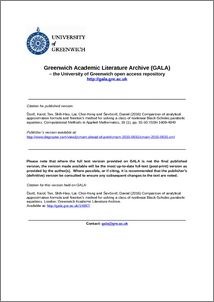# Comparison of analytical approximation formula and Newton's method for solving a class of nonlinear Black-Scholes parabolic equations

Ďuriš, Karol, Tan, Shih-Hau, and Ševčovič, Daniel (2016) Comparison of analytical approximation formula and Newton's method for solving a class of nonlinear Black-Scholes parabolic equations. Computational Methods in Applied Mathematics, 16 (1). pp. 35-50. ISSN 1609-4840 (Print), 1609-9389 (Online) (doi:https://doi.org/10.1515/cmam-2015-0035)Preview
PDF (Publisher PDF)
14897_Lai_Comparison_of_analytical_approximation_(Pub_PDF)_2015.pdf - Published Version

## Abstract

Market illiquidity, feedback effects, presence of transaction costs, risk from unprotected portfolio and other nonlinear effects in PDE-based option pricing models can be described by solutions to the generalized Black–Scholes parabolic equation with a diffusion term nonlinearly depending on the option price itself. In this paper, different linearization techniques such as Newton’s method and the analytic asymptotic approximation formula are adopted and compared for a wide class of nonlinear Black–Scholes equations including, in particular, the market illiquidity model and the risk-adjusted pricing model. Accuracy and time complexity of both numerical methods are compared. Furthermore, market quotes data was used to calibrate model parameters.

Item Type: Article Nonlinear PDE, Asymptotic Formula, Newton's Method, Finite Difference Method, Option Pricing, Black–Scholes Equation Faculty of Engineering & Science > School of Computing & Mathematical Sciences (CMS) 30 Apr 2020 10:16 http://gala.gre.ac.uk/id/eprint/14897

### Actions (login required)View Item